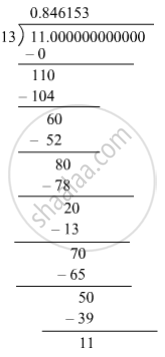SSC (English Medium) Class 8Maharashtra State Board
Share

# Write the Following Rational Number in Decimal Form. − 11 13 - SSC (English Medium) Class 8 - Mathematics

ConceptConcept for Decimal Representation of Rational Numbers

#### Question

Write the following rational number in decimal form.

-11/13

#### Solution

The given number is -11/13 .therefore 11/13 = 0.846153846153... = 0.bar846153

"The decimal form of " -11/13  "is"  -0.bar846153 .

Is there an error in this question or solution?

#### APPEARS IN

Balbharati Solution for Balbharati Class 8 Mathematics (2019 to Current)
Chapter 1: Rational and Irrational numbers
Practice Set 1.3 | Q: 5 | Page no. 4
Solution Write the Following Rational Number in Decimal Form. − 11 13 Concept: Concept for Decimal Representation of Rational Numbers.
S